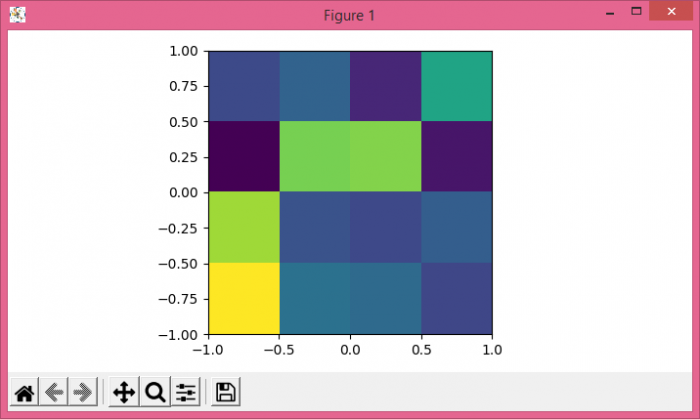# How to use 'extent' in matplotlib.pyplot.imshow?

To use extent in matplotlib imshow(), we can use extent [left, right, bottom, top].

## Steps

• Create random data using numpy.
• Display the data as an image, i.e., on a 2D regular raster with data and extent [−1, 1, −1, 1] arguments.
• To display the figure, use show() method.

## Example

import numpy as np
from matplotlib import pyplot as plt
plt.rcParams["figure.figsize"] = [7.00, 3.50]
plt.rcParams["figure.autolayout"] = True
data = np.random.rand(4, 4)
plt.imshow(data, extent=[-1, 1, -1, 1])
plt.show()

## Output